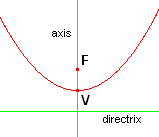www.batmath.it

A Glossary of Mathematical Terms - P

Palindrome

A number wich is the same left to right and right to left: 34543.

ParabolaOne of the conic sections.
V: vertex;
F: Focus.

Parallelepiped

A solid whose faces are all parallelograms.

Parallelogram

A quadrilateral with opposite sides that are parallel.

Parenthesis (pl.parentheses) ( ) (round brackets)

Simbols used, for example, in algebraic expressions.

A 15-sided polygon.

Pentagon

A five-sided polygon.

Percent

Part of 100.
Percent to decimal: 34% = .45;
Decimal to percent: 2.7 = 210%.

Percentile

A point that divides a set of data (arranged in ascending or descending order) into two parts: about n% of the data lie before the nth percentile, the rest is above it. The 50th percentile is also called the 2nd quartile.

Perfect square

A whole number that is the square of an integer.

Pie chart

See Circle graph.

Place value

It refers to the value of each poisiton or place in a number. In 3245 3 is in the thousands place.
Names of places values: ... billions (109), hundred millions (108), ten millions (107), millions (106), hundred thousands (105), ten thousands (104),  thosands (103), hundreds (102), tens (101), ones (100), tenths (10-1), hundredths (10-2), thosandths (10-3), ten thousandths (10-4), ...

Plane of symmetry

A plane taht divides a 3-D object into 2 parts, each a mirror image of the other.

Polarity of a variable

The positivity or negativity of the variable.

Polygon

A closed 2-D figure made up of line segments (at least 3).

Polyhedron

A 3-D object that has polygons as its faces. The intersection of any two faces is an edge.

Polynomial

An expression consisting of the sum of one or more monomials.

Power

A power: ab; a is the bases, b is the exponent (sometimes b itself is called the power).

Prime number

An integer greater than 1 whose only positive factors ar 1 and the number itself.

Prism

A solid with two congruent polygons as bases in parallel planes. All other faces are parallelograms. It is named according to the shape of its bases.

Product

See Multiplication.

Product set

The set of all couples formed by pairing every member of one set with every member of another set.

See Fraction.

Proper subset

A subset different from the null set and the parent set.

Proportion

An equality of two ratios: a : b = c : d.

Protractor (circular or semicircular)

A circular (or semicircular) device subdivided into 360 (or 180) equal parts, and used for measuring angles.

Pyramid

A solid with a polygon as base and all other faces triangular with a common vertex.

Pythagorean triple

A set of three natural numbers that can be the sides of a polygon.

first published on september 01 2003 - last updated on september 01 2003